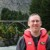# Which Statistical Test? Association or Correlelation?

Six Sigma – iSixSigma Forums General Forums General Which Statistical Test? Association or Correlelation?

Viewing 4 posts - 1 through 4 (of 4 total)
• Author
Posts
• #55947Vijay Narayan
Participant

Hi,
For a study in Uganda, I’m struggling to figure out a good way to measure association and/or correlation between 2 variables (continuous dependent variable, and dichotomous categorical independent variable).

The study looks at the relationship between ‘amount of time (# days) it takes for a patient’s blood sample to be processed by the laboratory’ and ‘whether or not the patient actually receives the test/result (yes/no)’. The null hypothesis is that there is no association between # of days to process blood sample in the lab and whether or not patient receives the test result.

So it is “whether patient receives his/her test results” (dichotomous categorical variable, independent) as a function of “blood sample processing time” (continuous variable, dependent).

The data is from 422 patients (for each, I have the # days and whether the patient received results). I believe the central limit theorem applies for distribution for sample processing time data points (# days).

Do you know what statistical test and measure would be most appropriate to assess association and correlation for this situation? Also, is association or correlation the better measure to use?

Many thanks!

0
#202310Robert Butler
Participant

The method of choice would be logistic regression, however, you have the variables flipped. The dependent variable is the receipt of the test result – the independent is the time it takes to process the sample. If you express it your way it doesn’t make any sense – if a person doesn’t have a blood sample taken then you can’t get a test result.

With logistic regression the output will be an odds ratio. In this instance you will want to use the “Yes Result” as the reference and predict the odds of a “No Result” as a function of elapsed days. Since time is a continuous variable the odds ratio will express the odds of a “No result” as a function of your unit of time.

So, for example, if the odds ratio was say 2.5 and the time unit was days it would say that the odds of not receiving a test result would increase 2.5 times for each day required to test the sample.

You can, of course, use “No Result” as a reference but, based on your description of the problem, this will result in odds ratios with values less than one. The interpretation is not that difficult but many people have a hard time understanding odds ratios when they are less than one.

So, if you use “No Result” as the reference and you get an odds ratio of say .75 then what that is telling you is that for each day required to process the sample the odds that the patient will receive a “Yes Result” decreases by 25%.

Now a comment: You statement concerning the central limit theorem makes no sense. The central limit theorem applies to the distribution of averages – not to the distribution of individual data points. In this instance, even if we were discussing averages, it would have no bearing on the situation.

0
#202311Chris Seider
Participant

Consider doing a box plot of days on Y axis versus result on X axis–will powefully demonstrate what the p-value says.

0
#202312Chris Seider
Participant

err powerfully :)

0
Viewing 4 posts - 1 through 4 (of 4 total)

You must be logged in to reply to this topic.